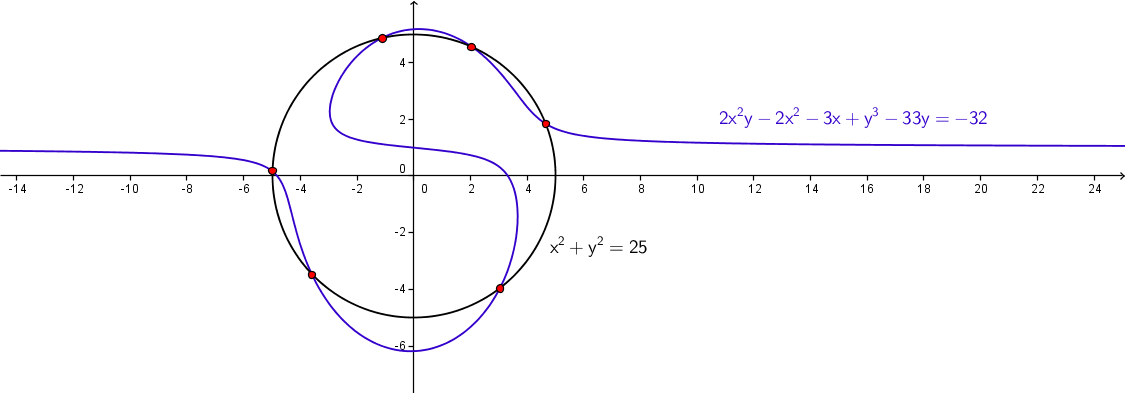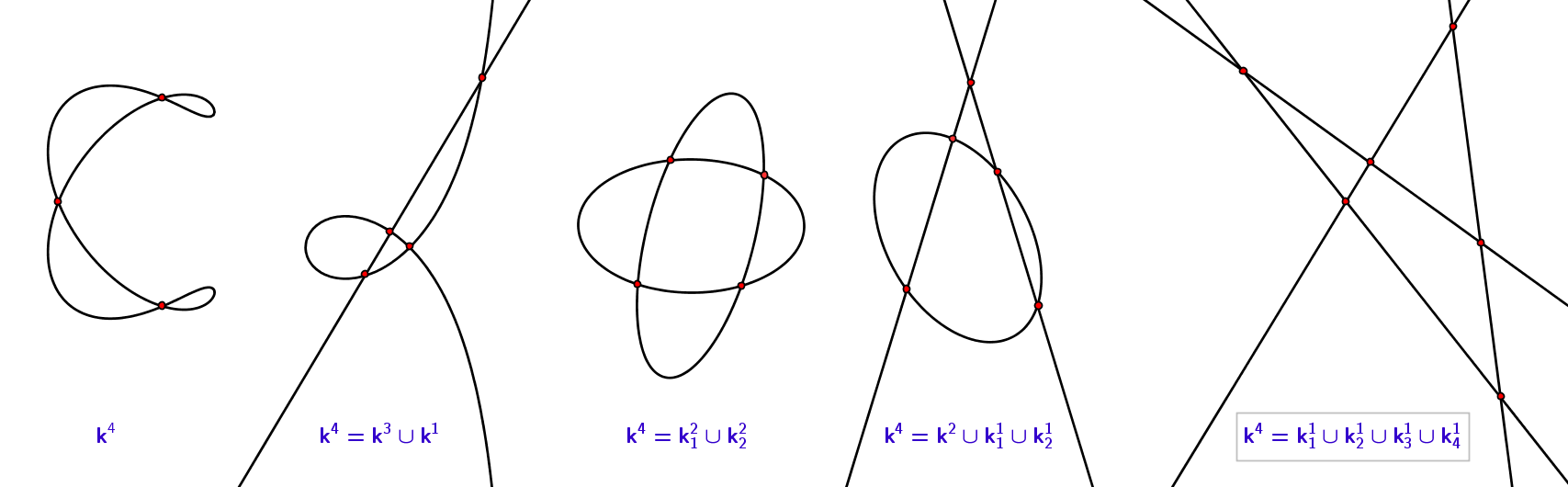## Plane algebraic curves

A plane curve is a set of  1 continuously connected points of a plane (set of dimension 1) .
There are numerous ways to continuously connect points, but we will concern only the curves that are defined by some algebraic equation.

A plane algebraic curve of degree n is a set of points T(x,y) whose coordinates satisfy an algebraic equation
f n(x,y)=0, where f n(x,y) is a polynomial of degree n.
As an example, equation 2x2y+y3−2x2−3x−33y+32=0 defines the blue curve of order 3 in Figure 1.

Since the system of two algebraic equations of degrees n and m with two indeterminates has n∗m solutions, two algebraic plane curves kn and km have n∗m common points which we call their intersection points. These points can be imaginary, in which case there is always a pair of conjugate points. These points can also coincide - in that case they are real points, and are counted multiply.

Figure 1 shows 6 real and different intersection points of a  3rd order curve and a circle given by the equation x2+y2−25=0.Figure 1

Since a line is a 1st order curve (given by a linear algebraic equation ax+by+c=0), the number of its intersection points with a plane algebraic curve of order n equals n. We use this fact to give an alternate geometric definition of the order of a curve:

Order of the plane algebraic curve equals the number of intersection points of this curve and any line that lies in the plane of this curve.

As was already mentioned, these intersection points may be conjugate imaginary points and as such impossible to be graphically represented in the real plane. If the intersection points are real and they coincide, two cases are possible. The intersection point is a double point of the curve, or it is a regular point at which the given line touches the curve. In the later, the line is called the tangent of  the curve.

Figure 2

Algebraic curves are sets of ∞1 continuously connected points. If a line intersect the curve in two infinitely close real points, we say this line is a tangent.  The point that contains these two infinitely close intersections of the tangent and the curve is called point of tangency. A line that passes through the point of tangency and is perpendicular to the tangent is called a normal line. If a curve has real intersections with the line at infinity, than the tangent line whose point of tangency is this point at infinity is called an asymptote.

Figure 3

A point on the curve that has a unique tangent line is called regular point of the curve. Almost all points of a plane algebraic curve are regular. There are singular points - points in which the curve has more than one tangent line. Examples of singular points are double points - points in which the curve intersects itself and in such points there are two different tangent lines. Number of double points on a curve is bounded: plane algebraic curve of order n can have at most (n−1)(n−2)/2 double points. If the number of double points is greater than this bound, then the curve is decomposed into curves of lower order so that the sum of their orders equals n.

Figure 4 shows one real (nondegenerate) curve of order 4 with 3 double points, and four cases of decomposition of a 4th order curve.Figure 4

Created by Sonja Gorjanc, translated by Helena Halas and Iva Kodrnja - 3DGeomTeh - Developing project of the University of Zagreb, made with GeoGebra